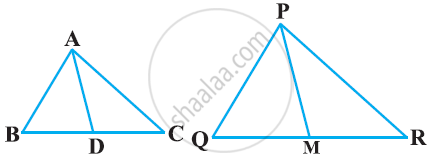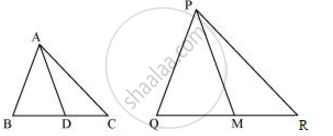# Sides AB and BC and median AD of a triangle ABC are respectively proportional to sides PQ and QR and median PM of ΔPQR (see the given figure). Show that ΔABC ∼ ΔPQR. - Mathematics

Sides AB and BC and median AD of a triangle ABC are respectively proportional to sides PQ and QR and median PM of ΔPQR (see the given figure). Show that ΔABC ∼ ΔPQR.#### SolutionMedian divides the opposite side.

:. BD = (BC)/2 and QM = (QR)/2

Given that

(AB)/(PQ) = (BC)/(QR)=(AD)/(PM)

=>(AB)/(PQ) = (1/2(BC))/(1/2QR) = (AD)/(PM)

=> (AB)/(PQ) = (BD)/(QM) = (AD)/(PM)

In ΔABD and ΔPQM,

(AB)/(PQ) = (BD)/(QM)= (AD)/(PM)  (Proved above)

∴ ΔABD ∼ ΔPQM (By SSS similarity criterion)

⇒ ∠ABD = ∠PQM (Corresponding angles of similar triangles)

In ΔABC and ΔPQR,

∠ABD = ∠PQM (Proved above)

(AB)/(PQ) = (BC)/(QR)

∴ ΔABC ∼ ΔPQR (By SAS similarity criterion)

Concept: Criteria for Similarity of Triangles
Is there an error in this question or solution?

#### APPEARS IN

NCERT Class 10 Maths
Chapter 6 Triangles
Exercise 6.3 | Q 12 | Page 141